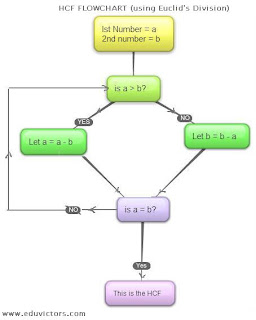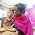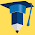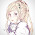## Friday, 12 April 2013

### CBSE Class 10 - Maths - Real Numbers (NCERT Ex 1.1)

Real Numbers
(NCERT Ex 1.1)

1. Use Euclid’s division algorithm to find the HCF of :

(i) 135 and 225
(ii) 196 and 38220
(iii) 867 and 255

Answer:
(i) 135 and 225
Step 1 : Since 225 > 135, apply Euclid’s division lemma to 225 and 135 to get
225 = 135 × 1 + 90

Step 2: Since remainder 90 ≠ 0, we apply the division lemma to 135 and 90 to get
135 = 90 × 1 + 45

Step 3: Now the remainder r = 45 ≠ 0 so we apply Euclid’s division lemma to 90 and 45 to have
90 = 2 × 45 + 0

Step 4: The remainder has now become zero. Since the divisor at this stage is 45,
∴ HCF(135,225) =45

(ii) 196 and 38220
Step 1: Since 38220 > 196, apply the division lemma to 38220 and 196 to get
38220 = 196 × 195 + 0

Step 2: Since remainder is zero, the process stops. Since the divisor at this stage is 196,
∴ HCF(38220, 196) = 196

(iii) 867 and 255

Step 1: Since 867 > 255, we apply the division lemma to 867 and 255 to get
867 = 255 × 3 + 102
Step 2: Since remainder 102 ≠ 0, we apply the division lemma to 255 and 102 to have
255 = 102 × 2 + 51
Step 3: Now the new divisor  is 102 and new remainder is 51. Apply the division lemma to get
102 = 51 × 2 + 0
Step 4: Since the remainder is zero, the process stops. Since the divisor at this stage is 51,
∴ HCF(867, 255) = 51

Q2: Show that any positive odd integer is of the form 6q + 1, or 6q + 3, or 6q + 5, where q is some integer.

Answer: Let a be any odd positive integer and b = 6.
By applying Euclid’s algorithm, we have
a = 6q + r          for some integer q ≥ 0, and r = 0, 1, 2, 3, 4, 5 because 0 ≤ r < 6.

∴ a = 6q or 6q + 1 or 6q + 2 or 6q + 3 or 6q + 4 or 6q + 5
Since a is an odd integer, it cannot take 6q, 6q + 2 and 6q + 4 as values.

Also, 6q + 1 = 2 × 3q + 1 = 2k1 + 1, where k1 is a positive integer
6q + 3 = (6q + 2) + 1 = 2 (3q + 1) + 1 = 2k2 + 1, where k2 is an integer
6q + 5 = (6q + 4) + 1 = 2 (3q + 2) + 1 = 2k3 + 1, where k3 is an integer

It is clear from above three statements that, 6q + 1, 6q + 3, 6q + 5 are of the form 2k + 1, where k is an integer.

Therefore, 6q + 1, 6q + 3, 6q + 5 are not exactly divisible by 2.
Hence, these expressions of numbers are odd numbers.
∴ any odd integer can be expressed in the form 6q + 1, or 6q + 3, or 6q + 5

Q3: An army contingent of 616 members is to march behind an army band of 32 members in a parade. The two groups are to march in the same number of columns. What is the maximum number of columns in which they can march?

Answer: HCF (616, 32) will give the maximum number of columns in which the Army contingent and the band can march.

Applying Euclid’s algorithm to find  HCF (616, 32),

Step 1: 616 = 32 × 19 + 8
Step2: 32 = 8 × 4 + 0
Step3: Since the remainder is zero, the divisor at this stage is 8. ∴ HCF (616, 32) = 8

Thus, they can march in 8 columns each.

Q4: Use Euclid’s division lemma to show that the square of any positive integer is either of the form 3m or 3m + 1 for some integer m.
[Hint: Let x be any positive integer then it is of the form 3q, 3q + 1 or 3q + 2. Now square each of these and show that they can be rewritten in the form 3m or 3m + 1.]

Answer: Let a be any positive integer and b = 3.
Applying Euclid's division lemma to a and b=3, we get

a = 3q + r for some integer q ≥ 0  and r = 0, 1, 2
⇒  a = 3q or 3q + 1 or 3q + 2
Squaring 'a' i.e.

a2 = (3q)2  or  (3q +1 )2  or (3q + 2)2
⇒  a2 = 9q2     or  (9q2 + 6q + 1 )  or (9q2 + 12q + 4)
⇒  a2 = 3(3q2)    or  3(3q2 + 2q ) + 1   or  3(3q2 + 4q + 1) + 1
⇒  a2 = 3 k1     or  3k2  + 1   or  3k3  + 1
where  k1= 3q2 ,  k2= 3q2 + 2q  and  k3=  3q2 + 4q + 1
⇒ Since q = 1,2,3... are integers. Their products and sums will also be integers. ∴ k1, k2, and k2 are also integers.

Thus we can say that the square of any positive integer is either of the form 3m or 3m + 1 for any integer m.

Q5: Use Euclid’s division lemma to show that the cube of any positive integer is of the form 9m, 9m + 1 or 9m + 8.

Answer: Let a be any positive integer and b = 3. Using Euclid’s division lemma we have
a = 3q + r, where q ≥ 0 and 0 ≤ r < 3
∴ a = 3q or 3q +1 or 3q + 2
Therefore, every number can be represented as these three forms. Therefore three cases arise:

Case 1: When a = 3q,
a3 = (3q)3 = 27q3 = 9(3q3) = 9m
Where m is an integer such that m =  3q3

Case 2: When a = 3q + 1,
a3 = (3q + 1)3
a3 = (27q3 + 27q2 + 9q + 1)  = 9(3q3 + 3q2 + q) + 1
a3 = 9m + 1
where m is an integer such that m = 3q3 + 3q2 + q

Case 3: When a = 3q + 2,
a3 = (3q + 2)3
a3 = (27q3 + 53q2 + 36q + 8)  = 9(3q3 + 6q2 + 4q) + 8
a3 = 9m +8
Where m is an integer such that m =  3q3 + 6q2 + 4q

Thus the cube of any positive integer is of the form 9m, 9m + 1, or 9m + 8.

Similar Questions asked in Examinations

Q6: Prove that 6 divides n(n + 1)(2n + 1) for any n ∈ N

Answer: If n = 1 or 2, ⇒ values of expression n(n + 1)(2n + 1) are 6 or 30 which are divisible by 6.
∴ n ≥ 3.
Also n and n+1 are consecutive numbers and either of them is an even number.
∴ n or n+1 is divisible by 2
or 2 divides  n(n + 1)(2n + 1)

Also n = 3k or 3k +1 or 3k + 2 for some k ∈ N

Case 1: when n = 3k,
n(n + 1)(2n + 1) = 3k(3k+1)(6k+1) which is divisible by 3

Case 2: when n = 3k + 1,
n(n + 1)(2n + 1)= (3k +1)(3k + 2)(6k + 3) = 3(3k +1)(3k + 2)(2k + 1) is divisible by 3.

Case 3: when n = 3k + 2,
n(n + 1)(2n + 1) = (3k + 2)(3k + 3)(6k +5) = 3(3k + 2)(k + 1)(6k +5) is divisible by 3.

∴ In all cases, 2 and  3 divides n(n +1)(2n + 1)
Since 2 and 3 have no common factor, thus we an say n(n + 1)(2n + 1)is divisible by 6.#### 11 comments:

1.2.For all cbse solutions and lectures....please visit dronstudy.com....!!!

3.i want more qustions of proving

4.ok for last question but should give more solved question

5.The flow chart to finding HCF is very good.

You can also watch these solutions in Video.

https://www.youtube.com/playlist?list=PLItJjtWTBUw6o6ZWYEfBNGnSlUZWD8mPY

1.Thank you so much!

6.not bad but i want more question for proving

7.not bad but i want more question for proving

8.9.In question 4 and 5,why are we taking 3q,3q+1,3q+2.....why not 2q,2q+1...or 5q,5q+1,5q+2 etc..??

10.In question 4 and 5,why are we taking 3q,3q+1,3q+2.....why not 2q,2q+1...or 5q,5q+1,5q+2 etc..??

We love to hear your thoughts about this post!